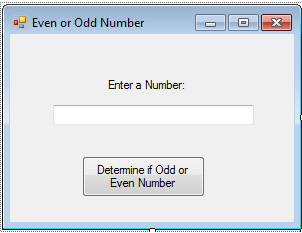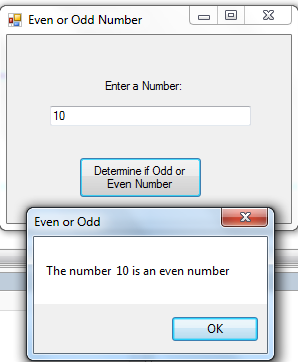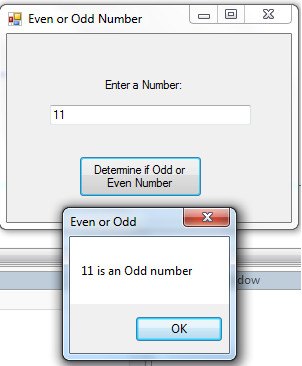# Determine Even or Odd Number in VB.NET Tutorial

Body

Even Numbers are any integer that can be divided exactly by 2. The last digit will be 0, 2, 4, 6, or 8. If it is not an even number, it is called an odd number. The last digit will be 1, 3, 5, 7, or 9.

In this tutorial, we will create a program that can determine a number whether odd or even.

Now, let's start this tutorial!

1. Let's start with creating a Windows Form Application for this tutorial by following the following steps in Microsoft Visual Studio: Go to File, click New Project, and choose Windows Application.

2. Next, add only one Button named Button1 and labeled it as "Determine if Odd or Even Number". Insert also Textbox and named it as Textbox1 for inputting the desired number. You must design your interface like this:3. Now, Copy and Paste the code below for your code module. The provided code is for the functionality of the "Button1_Click".

### Button Click function script:

`    Private Sub Button1_Click(ByVal sender As System.Object, ByVal e As System.EventArgs) Handles Button1.Click        Dim Mynumber As Integer        Dim isEven As Boolean        Mynumber = Val(TextBox1.Text)        If Mynumber Mod 2 = 0 Then            isEven = True            MsgBox("The number " & " " & Mynumber & " is an even number")        Else            MsgBox(Mynumber & " " & "is an Odd number")        End If     End Sub`

## Explanation:

We initialized variable "Mynumber" as Integer to hold the value inputted in "textbox1". The main reason to get the even number is to get their value as divisible by 2 which is equal to zero. So that is why we used the Mod function. Mod Operator divides two numbers and returns only the remainder. The number inputted in textbox1 was divided by 2 and if the remainder is zero then we considered it as an even number. Otherwise, it is an odd number in our If-Else statement.

### Output:For more inquiries and need programmer for your thesis systems in any kind of programming languages, just contact my number below.

Best Regards,

Engr. Lyndon R. Bermoy
IT Instructor/System Developer/Android Developer
Mobile: 09079373999
Telephone: 826-9296
E-mail:[email protected]

Visit and like my page on Facebook at: Bermz ISware Solutions

Subscribe at my YouTube Channel at: SerBermz

Visit my website at: CampCodes